# R S Aggarwal Solutions for Class 11 Maths Chapter 26 Three Dimensional Geometry Exercise 26C

R S Aggarwal Solutions for Class 11 Maths are given here for Chapter 26 exercise 26C. This exercise of RS Aggarwal Solutions is based on section formula, which states that,

The coordinates of point R that divide the line segment joining points A(x1, y1, z1) and B(x2, y2, z2) in the ratio m: n are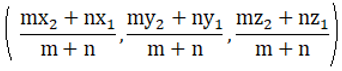Check the detailed R S Aggarwal solutions for class 11 chapter 26 exercise 26C below.

## Download PDF of R S Aggarwal Solutions for Class 11 Maths Chapter 26 Three Dimensional Geometry Exercise 26C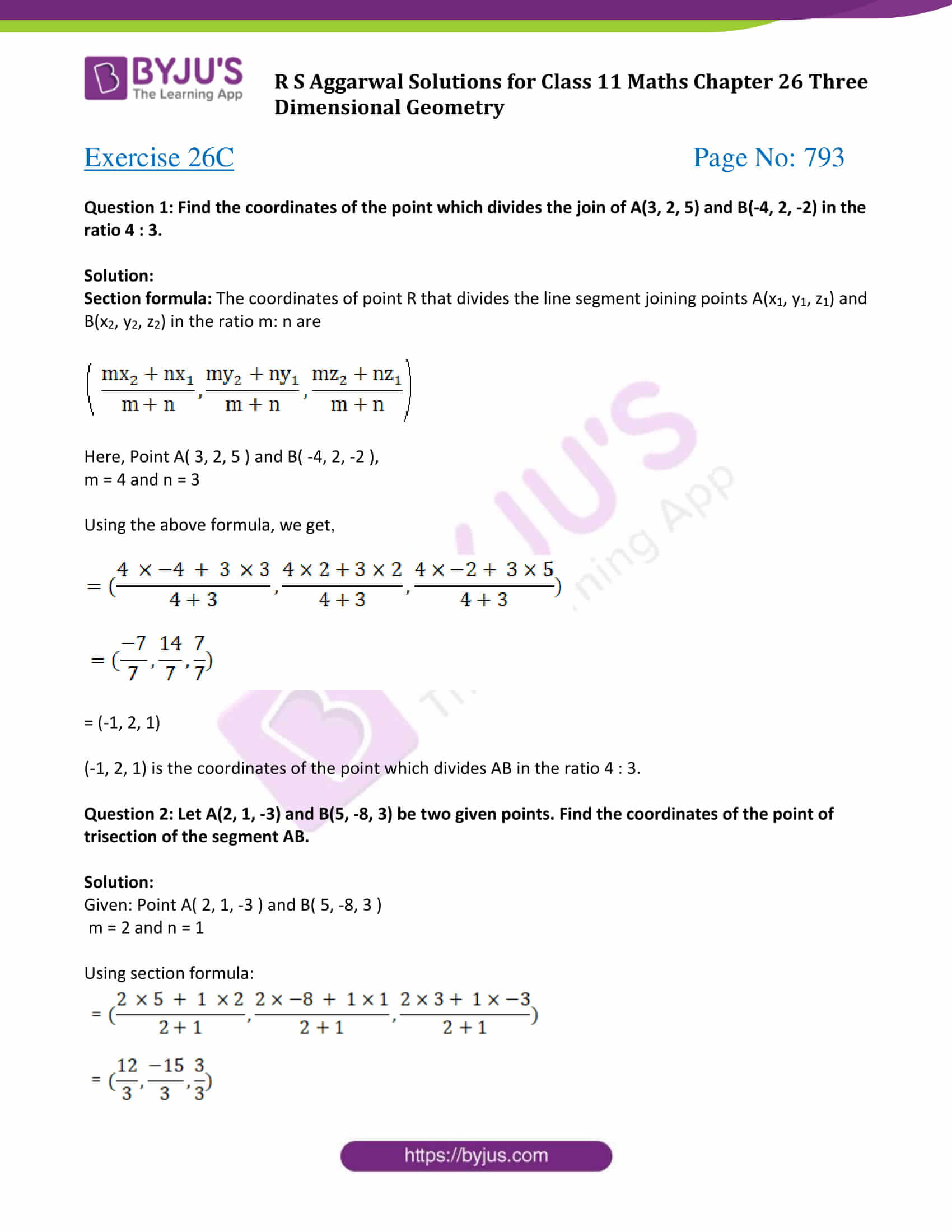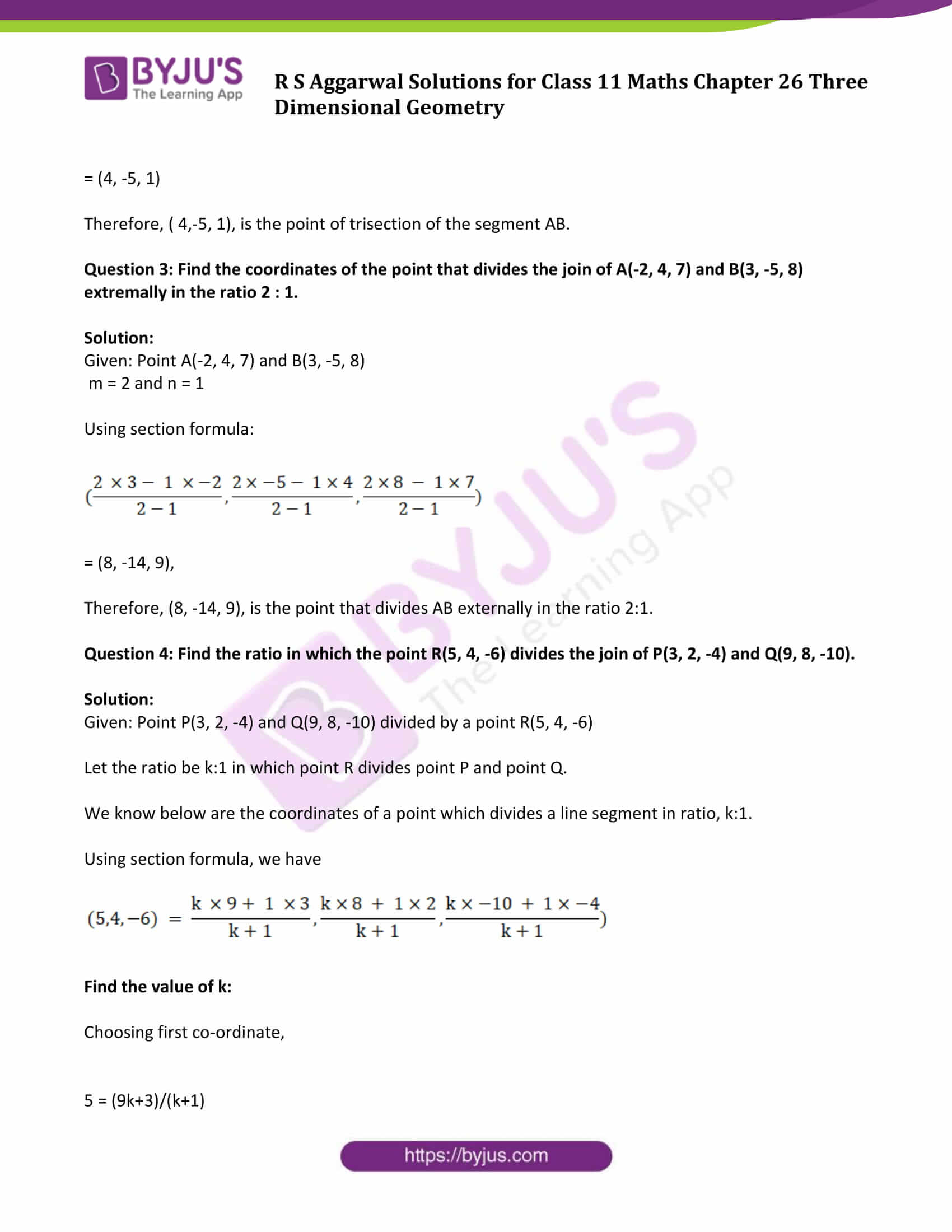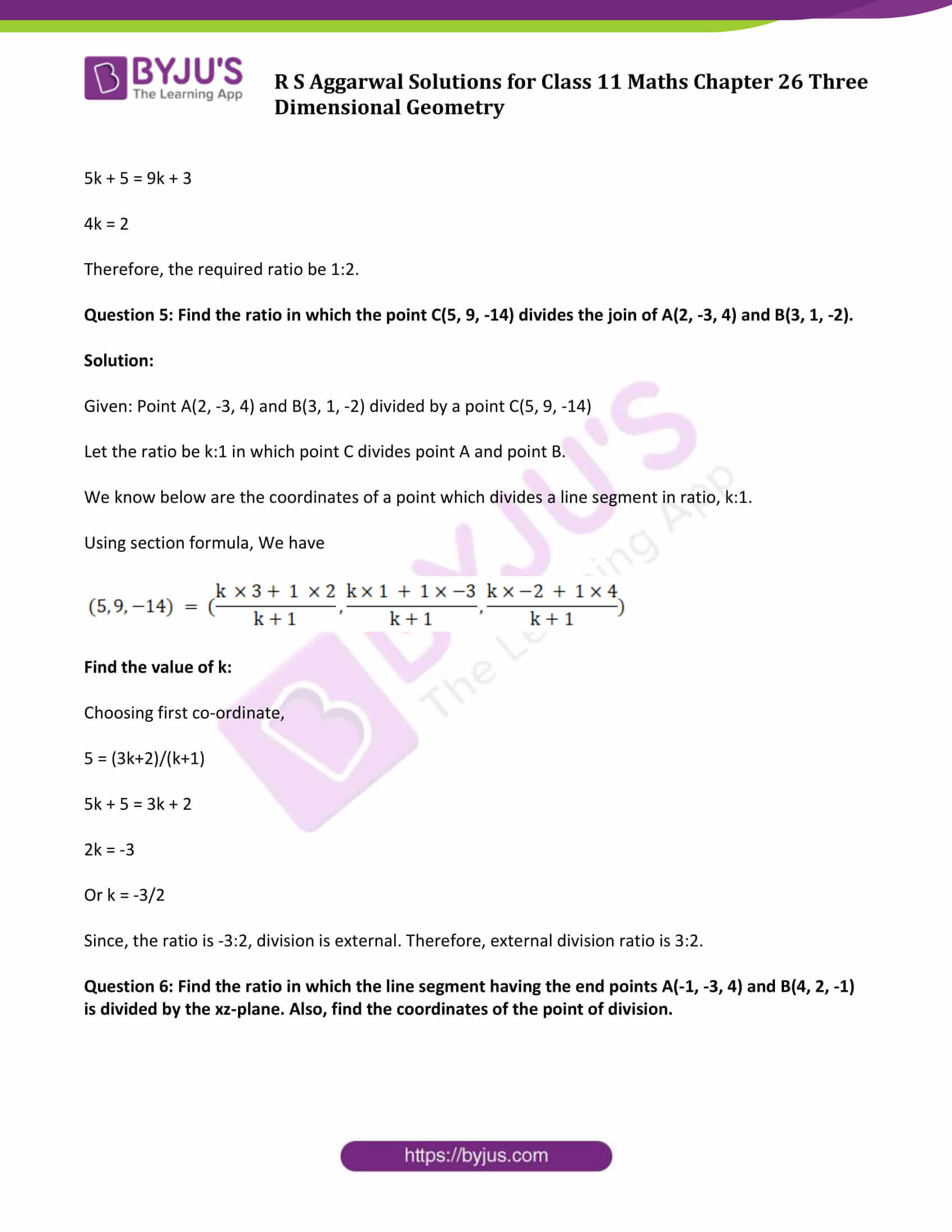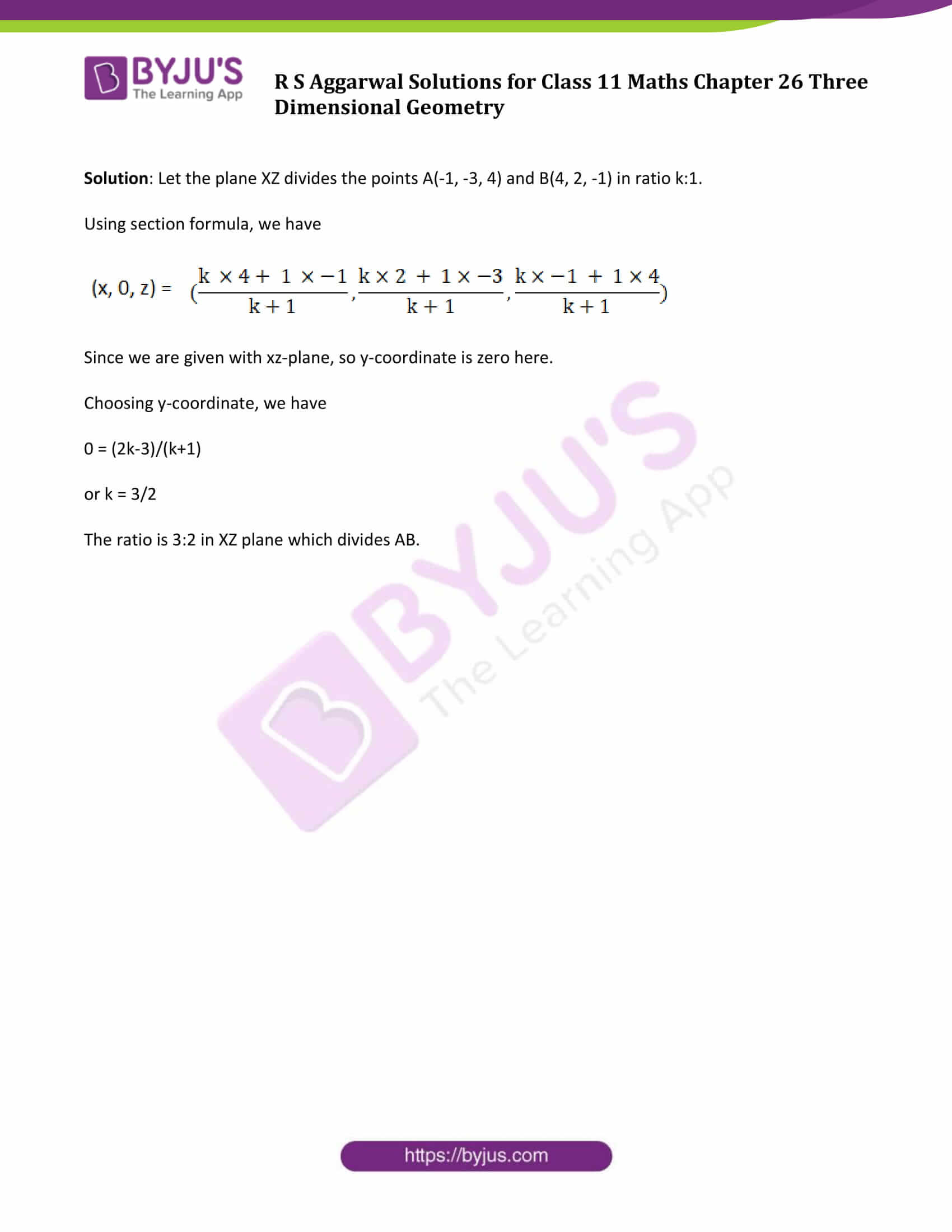### Access Answers of R S Aggarwal Solutions for Class 11 Maths Chapter 26 Three Dimensional Geometry Exercise 26C Page number 793

Exercise 26C Page No: 793

Question 1: Find the coordinates of the point which divides the join of A(3, 2, 5) and B(-4, 2, -2) in the ratio 4 : 3.

Solution:

Section formula: The coordinates of point R that divides the line segment joining points A(x1, y1, z1) and B(x2, y2, z2) in the ratio m: n are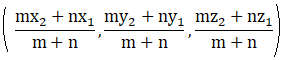Here, Point A( 3, 2, 5 ) and B( -4, 2, -2 ),

m = 4 and n = 3

Using the above formula, we get,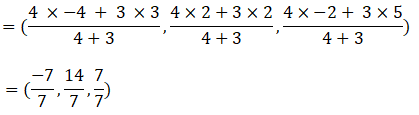= (-1, 2, 1)

(-1, 2, 1) is the coordinates of the point which divides AB in the ratio 4 : 3.

Question 2: Let A(2, 1, -3) and B(5, -8, 3) be two given points. Find the coordinates of the point of trisection of the segment AB.

Solution:

Given: Point A( 2, 1, -3 ) and B( 5, -8, 3 )

m = 2 and n = 1

Using section formula: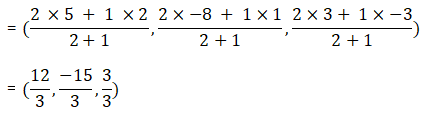= (4, -5, 1)

Therefore, ( 4,-5, 1), is the point of trisection of the segment AB.

Question 3: Find the coordinates of the point that divides the join of A(-2, 4, 7) and B(3, -5, 8) extremally in the ratio 2 : 1.

Solution:

Given: Point A(-2, 4, 7) and B(3, -5, 8)

m = 2 and n = 1

Using section formula: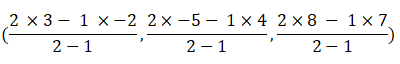= (8, -14, 9),

Therefore, (8, -14, 9), is the point that divides AB externally in the ratio 2:1.

Question 4: Find the ratio in which the point R(5, 4, -6) divides the join of P(3, 2, -4) and Q(9, 8, -10).

Solution:

Given: Point P(3, 2, -4) and Q(9, 8, -10) divided by a point R(5, 4, -6)

Let the ratio be k:1 in which point R divides point P and point Q.

We know below are the coordinates of a point which divides a line segment in ratio, k:1.

Using section formula, we have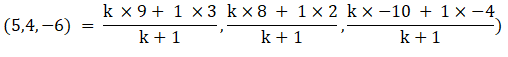Find the value of k:

Choosing first co-ordinate,

5 = (9k+3)/(k+1)

5k + 5 = 9k + 3

4k = 2

Therefore, the required ratio be 1:2.

Question 5: Find the ratio in which the point C(5, 9, -14) divides the join of A(2, -3, 4) and B(3, 1, -2).

Solution:

Given: Point A(2, -3, 4) and B(3, 1, -2) divided by a point C(5, 9, -14)

Let the ratio be k:1 in which point C divides point A and point B.

We know below are the coordinates of a point which divides a line segment in ratio, k:1.

Using section formula, We have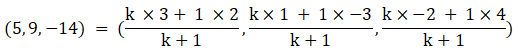Find the value of k:

Choosing first co-ordinate,

5 = (3k+2)/(k+1)

5k + 5 = 3k + 2

2k = -3

Or k = -3/2

Since, the ratio is -3:2, division is external. Therefore, external division ratio is 3:2.

Question 6: Find the ratio in which the line segment having the end points A(-1, -3, 4) and B(4, 2, -1) is divided by the xz-plane. Also, find the coordinates of the point of division.

Solution: Let the plane XZ divides the points A(-1, -3, 4) and B(4, 2, -1) in ratio k:1.

Using section formula, we have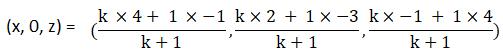Since we are given with xz-plane, so y-coordinate is zero here.

Choosing y-coordinate, we have

0 = (2k-3)/(k+1)

or k = 3/2

The ratio is 3:2 in XZ plane which divides AB.

## Access other exercise solutions of Class 11 Maths Chapter 26 Three Dimensional Geometry

Exercise 26A Solutions

Exercise 26B Solutions

## R S Aggarwal Solutions for Class 11 Maths Chapter 26 Exercise 26C

Class 11 Maths Chapter 26 Three Dimensional Geometry Exercise 26C is based on the following topics:

• Section formula
• Centroid of a triangle

In this exercise, students will get a chance to solve various problems on section formula and its applications such as they can check if the points are collinear or not and many more.